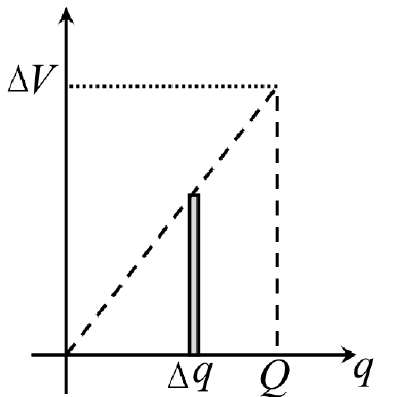Teaching Physics with the Physics Suite Edward F. Redish Problems Sorted by Type | Problems Sorted by Subject | Problems Sorted by Chapter in UP

The energy stored in a capacitor

 A capacitor stores a separation of charge. Since to separate the charges on a capacitor, you have to move charges against where the E field wants to push them, charging takes work. That work becomes stored energy -- just like carrying water up a hill so that you can let it roll down at a later time and turn a generator. A. Suppose the charges on the capacitor plates are equal to + q and - q . How much work does it take to carry a small positive charge Δq from the negative plate to the positive one, thereby increasing the charge on the plates? B. If you start with no charge on the plates and start to carry small charges over a bit at a time, the total charge Q will increase.   Since the potential difference is proportional to the charge on each plate, the graph of the potential difference vs. the amount of charge on the plate will be a straight line, as shown by the dotted line on the graph at the right.   From the geometry of this figure, find an expression for the total amount of work it takes to charge the plates up to a value of + Q, -QC. A 0.1 Farad capacitor (1 Farad = 1 Coulomb/Volt) is charged to 20 V and then connected in series to a 100 Ohm resistor.   Estimate for how much time current will flow through the resistor, starting from when the switch is connected.

Not finding what you wanted? Check the Site Map for more information.